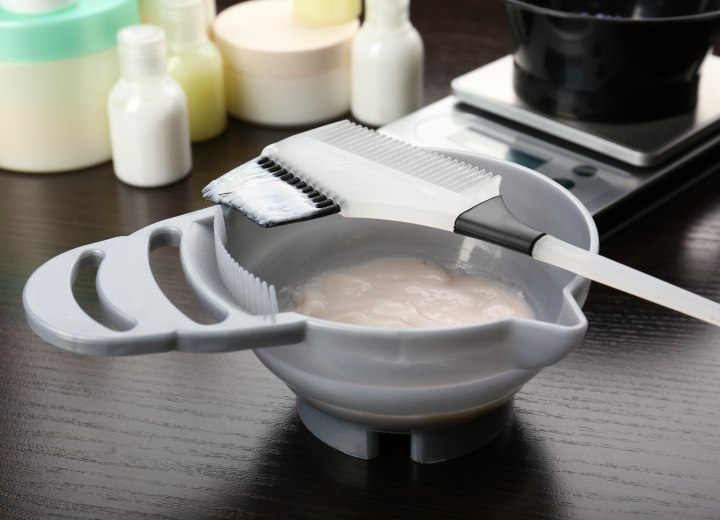# Formula to Mix PeroxideQ: I just completed Barber School and have already received my license. I have a question about peroxide. I had notes on this, but can't seem to find them and the information is not in my text book.

Ok, I was told that if I mixed a 10V with say a 40V I would get a 30V. They gave us a formula on how to mix different volumes with each other to come up with different strengths. Do you know the formula?

A: Well, it's actually simple mathematic equations. You are dealing with differing strengths of formula in specific quantities of volume, and you have to keep both the quantity and the strength in mind.

Let's start simply: Say you want to do a color process that only needs a little lift and you would normally use 20-volume peroxide, but all you have on hand is 40-volume peroxide. The 40-volume is too strong and would lighten the starting color too much. So you need some way to weaken the peroxide. To achieve this, you would need to combine the 40-volume peroxide with an equal amount of a neutral mixture (such as a cream conditioner).

So, you combine 1 oz. of 40-volume peroxide with 1 oz. of cream conditioner, and end up with 2 oz. of a mixture that is the equivalent to 20-volume peroxide. You have diluted the 40-volume developer by doubling the volume with a neutral product. The formula for what happened looks something like this: 1 oz. @ 40V + 1 oz. @ 0V = {40V + 0V}/2 oz. or 20V/oz.

Basically, each unit of peroxide must be counted. And by mixing and matching you can make varying strengths. For simplicity's sake let's use one-ounce (1 oz.) increments. Here are some examples:

If you have only 50V peroxide and 20V peroxide, you can achieve the following:

Combine 1 oz. 50V with 2 oz. 20V [50V + 20V + 20V = 90V/3 oz.] to get three ounces of 30V developer.

Combine 2 oz. 50V with 1 oz. 20V [50V + 50V + 20V = 120V/3 oz.] to get three ounces of 40V developer.

Combine 1 oz. 20V with 1 oz. of a neutral product (conditioner) [20V + 0V = 20V/2 oz.] to get two ounces of 10V developer.

So, you see, even with only 20V and 50V developer available, you can create any strength of developer that you need, up to the maximum strength available to you.